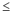# Specify how to estimate the parameters for Z-MR Chart

Stat > Control Charts > Variables Charts for Individuals > Z-MR > Z-MR Options > Estimate

## Method to define groups of observations

Minitab provides four methods for estimating the process standard deviations. You should choose an estimation method based on the properties of your particular process/product. You can also choose to enter a historical value in the Parameters dialog box.

• By runs: Use when you cannot assume all runs of a particular part or product have the same variance. This method estimates σ for each run independently.
• By parts (combine all observations for same part): Use when all runs of a particular part or product have the same variance. This method combines all runs of the same part or product to estimate σ.
• Constant (combine all observations): Use when all the output from your process has the same variance, regardless of the size of the measurement. This method pools all the data across runs and parts to obtain a common estimate of σ.
• Relative to size (combine all observations, use ln): Use when the variance increases in a fairly constant manner as the size of the measurement increases. This method takes the natural log of the data, pools the transformed data across all runs and all parts, and obtains a common estimate of s for the transformed data.

## Method for estimating standard deviation

Choose the method to estimate the standard deviation that Minitab uses to calculate the control limits.

Subgroup size = 1
• Average moving range: The average moving range is the average value of the moving range of two or more consecutive points. Use this method when the subgroup size is 1.
• Median moving range: The median moving range is the median value of the moving range of two or more consecutive points. Use this method when the data have extreme ranges that influence average of the moving ranges.

## Specify length of moving range

In Length of moving range, enter the number of observations that are used to calculate the moving range. By default, a length of 2 is used because consecutive values have the greatest chance of being alike. The length must be100.

If the data are cyclical, consider using a moving range of a different length. For example, if you collect quarterly data, consider using a moving range of length 4 to ensure that one observation from each season is included in the calculation.

By using this site you agree to the use of cookies for analytics and personalized content.  Read our policy